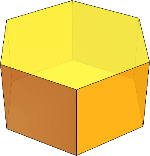Search IntMath
Close

450+ Math Lessons written by Math Professors and Teachers

5 Million+ Students Helped Each Year

1200+ Articles Written by Math Educators and Enthusiasts

Simplifying and Teaching Math for Over 23 Years

# What is a Hexagonal Prism in Geometry?A hexagonal prism is a 3D geometric shape that has six faces and eight vertices. It is a type of polyhedron, which is a 3D shape with flat faces and straight edges. The faces of a hexagonal prism are comprised of six rectangular sides and two hexagonal bases.

## The Components of a Hexagonal Prism

A hexagonal prism has eight vertices, twelve edges, and six faces. The edges are the straight lines that connect the vertices, and the faces are the flat surfaces of the prism. The vertices of a hexagonal prism are made up of three sets of four vertices each, with each set forming a regular hexagon.

The six faces of the hexagonal prism are all rectangles with different lengths. The two hexagonal bases of the prism have equal sides, but the rectangles have different lengths. The four rectangles on the sides of the prism are all the same length, whereas the two rectangles on the ends of the prism have different lengths.

## Properties of a Hexagonal Prism

A hexagonal prism is a three-dimensional shape, which means that it has length, width, and height. The length of the prism is the distance between the two hexagonal bases, and the width is the length of each side of the hexagonal bases. The height of the prism is the length of the four rectangles on the sides of the prism.

The two hexagonal bases of the hexagonal prism are parallel to each other, and the four rectangles on the sides of the prism are also parallel to each other. The diagonals of the hexagonal bases are all the same length, and the diagonals of the rectangles on the sides of the prism are also all the same length.

## How to Calculate the Volume of a Hexagonal Prism

The volume of a hexagonal prism is the total space inside the prism, and it can be calculated by multiplying the area of the hexagonal bases by the height of the prism. The area of each base is calculated by multiplying the length of one side of the hexagon by the length of the diagonal. The height of the prism is the length of the four rectangles on the sides of the prism.

## Practice Problems

1. Calculate the volume of a hexagonal prism with a base side length of 7 cm and a height of 10 cm.

Answer: The volume of the hexagonal prism is 490 cm3.

2. Calculate the length of the diagonals of the hexagonal bases of a hexagonal prism with a base side length of 5 cm.

Answer: The length of the diagonals of the hexagonal bases is 8.66 cm.

3. Calculate the area of one hexagonal base of a hexagonal prism with a base side length of 6 cm.

Answer: The area of one hexagonal base is 27.71 cm2.

4. Calculate the length of the four rectangles on the sides of a hexagonal prism with a height of 9 cm.

Answer: The length of the four rectangles on the sides of the prism is 9 cm.

5. Calculate the total number of edges of a hexagonal prism.

Answer: The total number of edges of a hexagonal prism is 12.

6. Calculate the total number of vertices of a hexagonal prism.

Answer: The total number of vertices of a hexagonal prism is 8.

## Summary

A hexagonal prism is a 3D geometric shape that has six faces and eight vertices. It is a type of polyhedron, which is a 3D shape with flat faces and straight edges. The faces of a hexagonal prism are comprised of six rectangular sides and two hexagonal bases. The volume of a hexagonal prism can be calculated by multiplying the area of the hexagonal bases by the height of the prism. The length of the diagonals of the hexagonal bases, the area of one hexagonal base, and the length of the four rectangles on the sides of the prism can also be calculated. The total number of edges and vertices of a hexagonal prism are 12 and 8, respectively.

In summary, a hexagonal prism is a 3D geometric shape with six faces and eight vertices. It has different properties, such as its length, width, and height, as well as the lengths of its diagonals, the area of its hexagonal bases, and the length of its four rectangles on the sides. The volume of a hexagonal prism can be calculated by multiplying the area of the hexagonal bases by the height of the prism. The total number of edges and vertices of a hexagonal prism are 12 and 8, respectively.

## FAQ

### What do you mean by hexagonal prism?

A hexagonal prism is a 3-dimensional shape with 8 faces, 18 edges, and 12 vertices. It consists of two hexagonal bases and six rectangular faces.

### What is an example of a hexagonal prism?

An example of a hexagonal prism is a beehive.

### What is a hexagon in geometry?

A hexagon is a 6-sided polygon. It has 6 angles, 6 sides, and 6 vertices.

### What is the figure of hexagonal prism?

The figure of a hexagonal prism is a 3-dimensional shape with two hexagonal bases and six rectangular faces.

## Problem SolverThis tool combines the power of mathematical computation engine that excels at solving mathematical formulas with the power of GPT large language models to parse and generate natural language. This creates math problem solver thats more accurate than ChatGPT, more flexible than a calculator, and faster answers than a human tutor. Learn More.